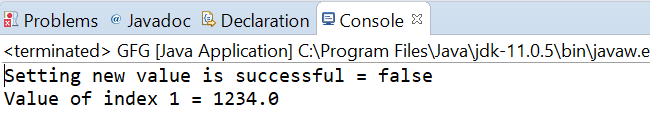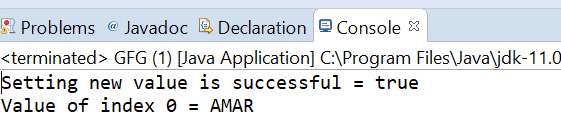# AtomicReferenceArray weakCompareAndSetVolatile() method in Java with Examples

• Last Updated : 03 Jan, 2020

The weakCompareAndSetVolatile() method of a AtomicReferenceArray class is used to atomically sets the value of the element at index i to newValue to newValue for AtomicReferenceArray if the current value is equal to expectedValue passed as parameter. This method updates the value with memory effects as specified by VarHandle.weakCompareAndSet(java.lang.Object…).This method returns true if set a new value to AtomicRefrence is successful.

Syntax:

```public final boolean
weakCompareAndSetVolatile(int i,
E expectedValue,
E newValue)
```

Parameters: This method accepts i which is an index of AtomicReferenceArray to perform the operation, expectedValue which is the expected value and newValue which is the new value to set.

Return value: This method returns true if successful.

Below programs illustrate the weakCompareAndSetVolatile() method:
Program 1:

 `// Java program to demonstrate AtomicReferenceArray``// weakCompareAndSetVolatile() method`` ` `import` `java.util.concurrent.atomic.AtomicReferenceArray;`` ` `public` `class` `GFG {``    ``public` `static` `void` `main(String[] args)``    ``{`` ` `        ``// create an atomic reference object.``        ``AtomicReferenceArray ref``            ``= ``new` `AtomicReferenceArray(``5``);`` ` `        ``// set some value``        ``ref.set(``1``, ``1234.00``);``        ``ref.set(``0``, ``2234.00``);``        ``ref.set(``2``, ``3234.00``);`` ` `        ``// apply weakCompareAndSetVolatile()``        ``boolean` `result``            ``= ref.weakCompareAndSetVolatile(``1``, ``124.00``,``                                            ``234.32``);`` ` `        ``// print value``        ``System.out.println(``"Setting new value"``                           ``+ ``" is successful = "``                           ``+ result);`` ` `        ``System.out.println(``"Value of index 1 = "``                           ``+ ref.get(``1``));``    ``}``}`

Output:Program 2:

 `// Java program to demonstrate AtomicReferenceArray``// weakCompareAndSetVolatile() method`` ` `import` `java.util.concurrent.atomic.AtomicReferenceArray;`` ` `public` `class` `GFG {``    ``public` `static` `void` `main(String[] args)``    ``{`` ` `        ``// create an atomic reference object.``        ``AtomicReferenceArray ref``            ``= ``new` `AtomicReferenceArray(``5``);`` ` `        ``// set some value``        ``ref.set(``0``, ``"AMANDIP"``);``        ``ref.set(``1``, ``"AMAN"``);`` ` `        ``// apply weakCompareAndSetVolatile()``        ``boolean` `result``            ``= ref.weakCompareAndSetVolatile(``                ``0``,``                ``"AMARDIP"``,``                ``"AMAR"``);`` ` `        ``// print value``        ``System.out.println(``"Setting new value"``                           ``+ ``" is successful = "``                           ``+ result);`` ` `        ``System.out.println(``"Value of index 0 = "``                           ``+ ref.get(``0``));``    ``}``}`

Output:My Personal Notes arrow_drop_up